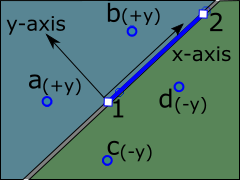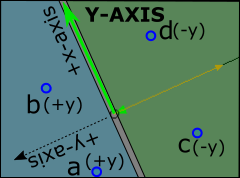﻿ MechDesigner Reference & User Interface > FAQs and 'How do I...?' > Part Editor: FAQs and How to...? > Why is the dimension 'negative' in the Dimension dialog'?

# Why is the dimension 'negative' in the Dimension dialog'?

### Why is my dimension negative?

#### Negative Dimensions.

The sign appears if the coordinate of the dimension is negative.

Generally, do not delete the sign, but edit the dimension in the normal way.

#### More DetailsLines have an X-Y coordinate-system. •The Origin of a Line is at its start-Point •The +X-axis of a Line is from its start-Point(1) to its end-Point(2) •The +Y-axis of a Line is at +90º from Line. When we add a dimension that is a perpendicular-distance from the Line, the •dimension will be Positive if in +y-axis half of the Line (blue area in the image) •dimension will be Negative if in the -y-axis of the Line (green area in the image) In the top image: •Dimensions from the Line(1 to 2) to Points a and b will be positive •Dimensions from the Line to Points c and d will be negativeThe X-AXIS and Y-AXIS of a Part each has its own coordinate-system. The image to the left shows the Coordinate-System of the Y-AXIS The local +x-axis of the Y-AXIS of a Part is along the Y-AXIS! Thus, the local +y-axis of the Y-axis of a Part is at +90º from Y-axis Thus a dimension that is a perpendicular-distance from the Y-AXIS to a Point is in the green area will be negative ... ... and this is in the +X axis direction of the Part!